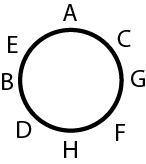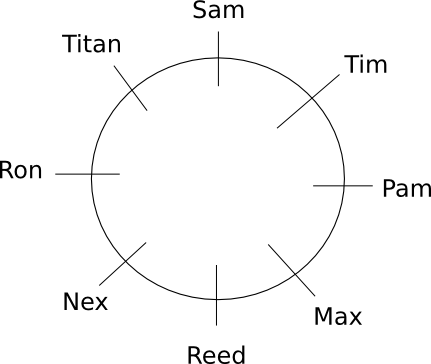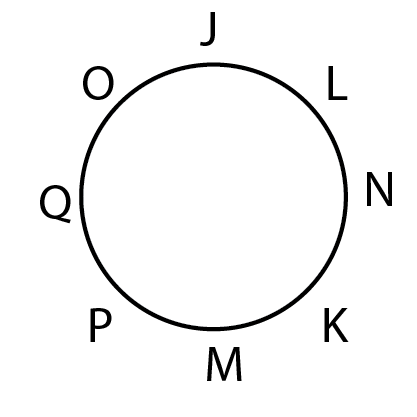# Seating Arrangement Questions for RRB Group-D set-2 PDF

0
3297

### Seating Arrangement Questions for RRB Group-D set-2 PDF

Download Top-15 RRB Group-D Seating Arrangement Questions set-2 PDF. RRB GROUP-D Reasoning questions based on asked questions in previous exam papers very important for the Railway Group-D exam.

Question 1: 22 boys are standing in front of Kunal in a queue. 12 boys are standing to the back of Rohit in the same queue. If total number of boys is 30 then the number of boys standing in between Kunal and Rohit is

a) 3

b) 4

c) 6

d) 5

Question 2: 32 boys are standing in front of X in a queue. Y is standing in the 19th position from the back in the same queue.If total number of boys is 45 then the number of boys standing in between X and Y is

a) 3

b) 5

c) 6

d) 4

Instructions

Eight people are sitting facing to the centre in a circular path. A ,H and B,G are at the diametrically opposite ends and they all are not immediate neighbours of each other. C,D are at the diametrically opposit positions and C is an immediate neighbour to G. F is also an immediate neighbour to G.

Question 3: Who is sitting between B and A?

a) F

b) G

c) H

d) E

Question 4: Who is sitting between D and F?

a) I

b) H

c) G

d) F

Question 5: What is the order of the people in clockwise direction starting from A?

a) BEDFHCGA

b) AGCHFDEB

c) ACGFHDBE

d) FGCAHDBE

Question 6: What letter will be at the 5th position starting from A in anticlockwise direction?

a) I

b) F

c) E

d) D

Instructions

A, B , C, D, E, F, G and H are standing in a queue from left to right. A is standing third to the left of D. H is standing second to the right of D. C is second to the right of B. Neither A nor D is standing next to C. G is third to the left of E.

Question 7: Who is standing at the extreme left end of the row?

a) A

b) G

c) C

d) D

Question 8: How many people are standing between G and C?

a) 3

b) 4

c) 5

d) 6

Question 9: How many people are standing between D and B?

a) 0

b) 1

c) 2

d) 3

Question 10: What is the position of A with respect to H?

a) fourth to the left

b) fifth to the right

c) fifth to the left

d) fourth to the right

Instructions

Use the following information to answer the questions.

Max, Titan, Nex, Sam, Ron, Tim, Pam and Reed are sitting around a circular table. All of them are facing the center. Tim is fourth to the right of Nex. Ron is fifth to the left of Tim. Max is third to the left of Sam. Max is not sitting next to Ron or Tim. Reed is third to the right of Titan. Reed and Ron are not sitting next to each other.

Question 11: Who is third to the right of Tim?

a) Nex

b) Pam

c) Reed

d) Ron

Question 12: Who is second to the left of Ron?

a) Sam

b) Reed

c) Titan

d) Max

Question 13: In which of the following pairs is the second person sitting to the immediate right of the first person?

a) Max, Tim

b) Sam, Titan

c) Reed, Pam

d) Ron, Sam

Question 14: Where is Tim sitting with respect to Reed?

a) third to the left

b) second to the right

c) third to the right

d) second to the left

Instructions

8 people are sitting in a circle facing to the center. J,M and N,Q are at the diametrically opposite positions and J,Q,M and N are not immediate neighbors. O and K are opposite to each other and L is an immediate neighbor to N.

Question 15: Who is sitting between K and P?

a) M

b) L

c) Q

d) O

22 boys are standing in front of Kunal in a Queue 12 boys are standing to the back of Rohit
Total boys = 30.
So, rank of Kunal in the queue is 22+1 = 23
Rank of Rohit in the queue is 30 – 12 = 18
Hence, the number of boys between Rohit and Kunal in the queue is 23 – 18 – 1 = 4

There are 32 boys ahead of X and 26 ahead of Y. Since Y is also ahead of X, there are 31 other boys ahead of X. ie there are 5 boys between X and Y.According to the given information, people are sitting in the anticlockwise direction as follows: A,E,B,D,H,F,G,C ;
Hence, E is sitting between B and AAccording to the given information, people are sitting in the anticlockwise direction as follows: A,E,B,D,H,F,G ;

The order of sitting in the clockwise direction will be c as ACGFHDBE: According to the given informations, people are sitting in the anticlockwise direction as follows: A,E,B,D,H,F,G,C ;The possibilities from the first two statements are:

A _ _ D _ H _ _ _ A _ _ D _ H _ _ _ A _ _ D _ H
Since C is not next to either A or D, the possibilities are:

A _ _ D _ H C _ , A _ _ D _ H C _ _ A _ _ D _ H C C _ A _ _ D _ H

From the other statements, the only possibility becomes G A F E D B H C

The order of arrangement from left to right is as follows: G A F E D B H C

So, G is standing at the extreme end of the row.

The possibilities from the first two statements are:

A _ _ D _ H _ _ _ A _ _ D _ H _ _ _ A _ _ D _ H
Since C is not next to either A or D, the possibilities are:

A _ _ D _ H C _ , A _ _ D _ H C _ _ A _ _ D _ H C C _ A _ _ D _ H

From the other statements, the only possibility becomes G A F E D B H C

The order of arrangement from left to right is as follows: G A F E D B H C

So, there are 6 people between G and C.

The possibilities from the first two statements are:

A _ _ D _ H _ _ _ A _ _ D _ H _ _ _ A _ _ D _ H
Since C is not next to either A or D, the possibilities are:

A _ _ D _ H C _ , A _ _ D _ H C _ _ A _ _ D _ H C C _ A _ _ D _ H

From the other statements, the only possibility becomes G A F E D B H C

The order of arrangement from left to right is as follows: G A F E D B H C

So, there are 0 people between B and D.

The possibilities from the first two statements are:

A _ _ D _ H _ _ _ A _ _ D _ H _ _ _ A _ _ D _ H
Since C is not next to either A or D, the possibilities are:

A _ _ D _ H C _ , A _ _ D _ H C _ _ A _ _ D _ H C C _ A _ _ D _ H

From the other statements, the only possibility becomes G A F E D B H C

The order of arrangement from left to right is as follows: G A F E D B H C

So, the position of A with respect to H is fifth to the left.

The arrangement of the 8 people around the circular table is as follows:So, the person who is third to the right of Tim is Ron.

The arrangement of the 8 people around the circular table is as follows:So, the person who is second to the left of Ron is Sam.

The arrangement of the 8 people around the circular table is as follows:So, Tim is sitting to the immediate right of Sam.

The arrangement of the 8 people around the circular table is as follows:So, Tim is sitting third to the right of Reed.